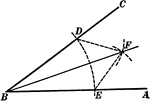### Construction Of Angle Bisector

Illustration showing how to construct the bisector of an angle.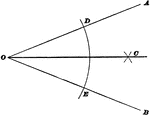### Bisect An Angle

Illustration used to show how to construct a bisector of an angle when the sides intersect within the…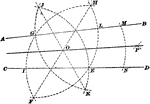### Bisect An Angle

Illustration used to show how to construct a bisector of an angle when the sides no not intersect within…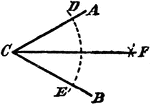### Construction Of A Divided Angle

An illustration showing the construction used to divide an angle into two equal parts. "With C as a…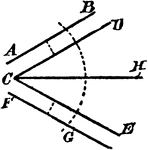### Construction Of A Divided Angle

An illustration showing the construction used to divide an angle into two equal parts when the lines…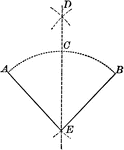### Construction of Bisecting a Given Angle

Illustration of the construction used to bisect a given angle.### Centers of Equilateral Triangle

Illustration of an equilateral triangle that shows both the centroid (where the medians of the sides…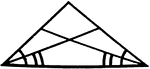### Angle Bisectors In An Isosceles Triangle

Illustration showing that if two angles of a triangle are equal, the bisectors of these angles are equal.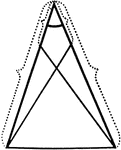### Equal Segments In An Isosceles Triangle

Illustration showing that if equal segments measured from the vertex are laid off on the arms of an…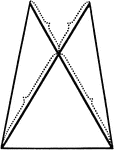### Equal Segments In An Isosceles Triangle

Illustration showing that if equal segments prolonged through the vertex are laid off on the arms of…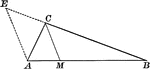### Angle Bisector of Triangle Dividing Opposite Side Into Proportionate Segments

Illustration to show that an angle bisector of a triangle divides the opposite side into segments which…### Exterior Angle Bisector of Triangle Dividing Opposite Side Into Proportionate Segments

Illustration to show that a bisector of a an exterior angle of a triangle divides the opposite side…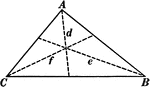### Angle Bisectors In A Triangle

Illustration showing the three angle bisectors in a triangle.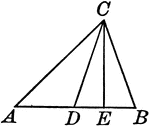### Triangle With Bisector and Perpendicular From Vertex Drawn

Illustration to show the bisector of the vertical angle of a triangle makes with the perpendicular from…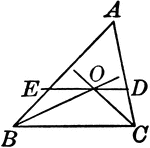### Triangle With Bisectors of Base Angles

Illustration to show if the bisector of the base angles of a triangle are drawn, and through their point…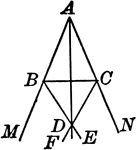### Triangle With Bisectors of Vertical Angles

Illustration to show the bisector of the vertical angle A of a triangle ABC, and the bisectors of the…